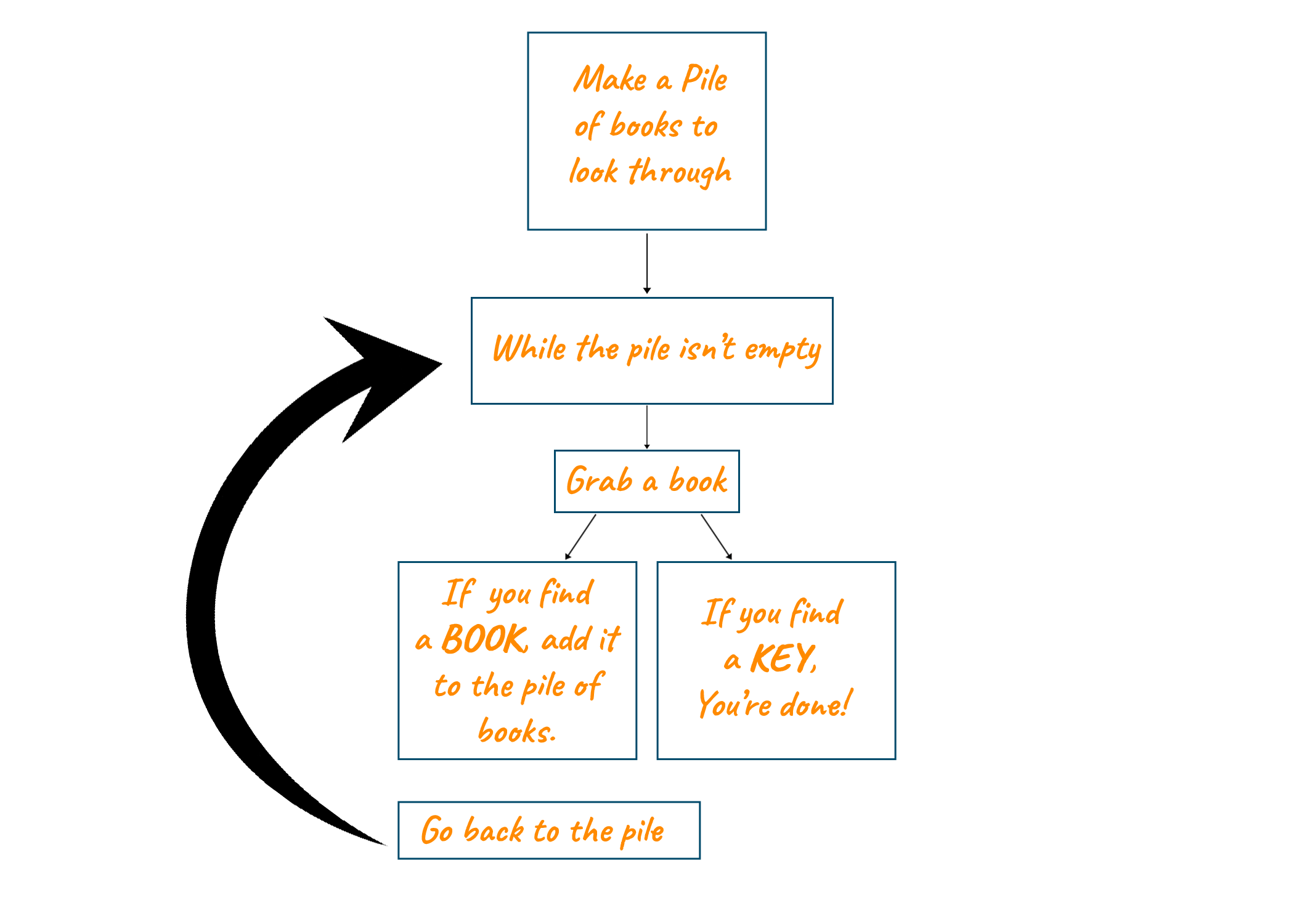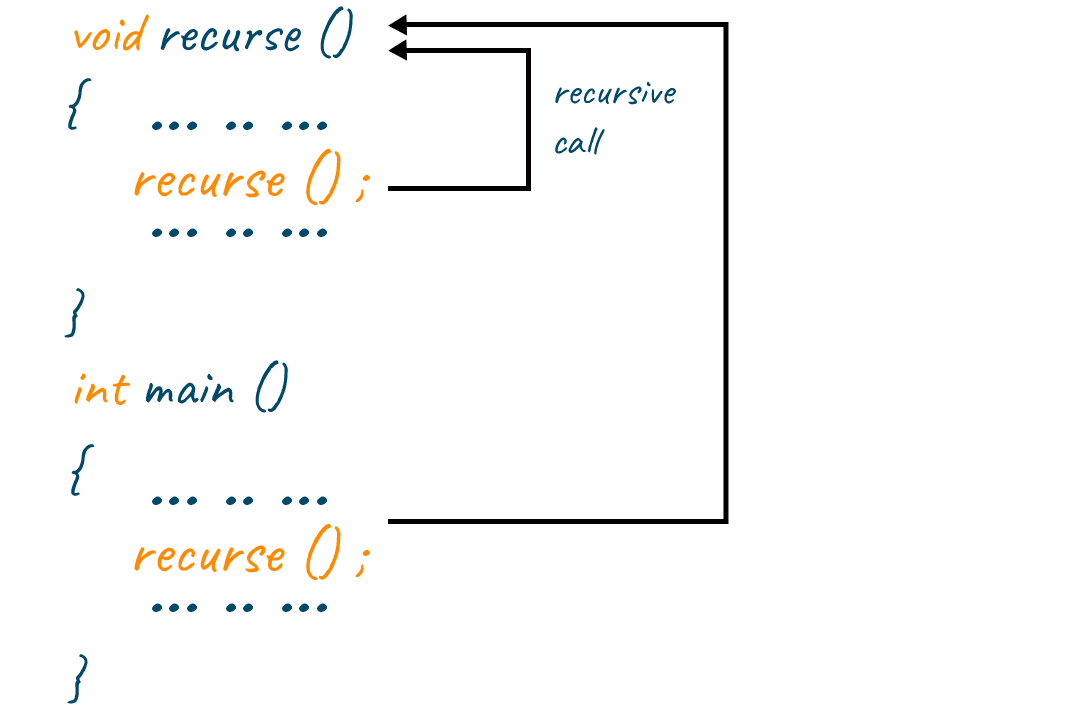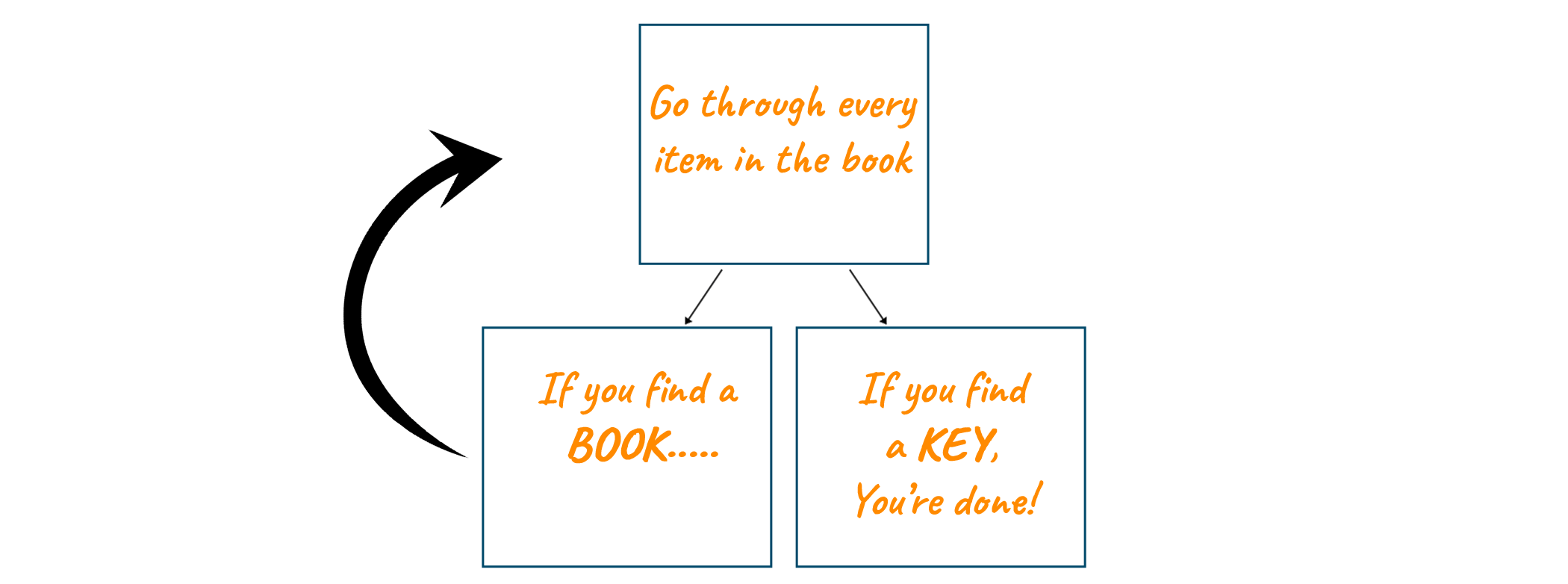# Recursion Vs Iteration |10 Differences (& When to use?)

Whenever we try to code two terms, namely iteration and recursion, often pop up. And for a newcomer, these two can be a little confusing. If this is the case for you, then do not worry as we are here to help you out. Both these terms refer to two different code structures with the same ultimate goal: repeated execution of a set of sequential instructions. In this article, we will study what is recursion and what is iteration, followed by a table comparing both of them. So, let’s get started.

## What is Iteration?

In this type of code, structure loops are used to execute a set of instructions. In other words, iteration code structure uses repetition structure. Any part of code which uses a loop is said to follow iteration code structure.

To help you visualize this concept, let us take an example of a pile of books, and a key is kept between any two books. Our aim is to find that key. So, what you do is you start picking the books one by one, and each time you pick a book you check a condition: whether you have found the key or not. If you have found the key that our job is done. However, if we haven’t then we continue to pick the books from the pile one by one until we reach the bottom. This sort of execution of a task is known as the Iteration model. Look at the flowchart below, to further solidify this concept.In Iteration the set of statements execute until the condition in the iteration statement is True. When the condition evaluates to be false, the iteration is said to terminate. At any point of time, the state of an iteration is defined by its control variable. A control variable is that variable that is responsible for running a loop ( generally i or j in most cases). In case of an absence of a condition the statement, the loop utilizes our CPU cycles and we enter into a condition known as an infinite loop.

The time complexity of a program following the iteration code structure can be easily calculated by counting the number of times the statements inside the loop are getting executed.

## What is Recursion?

Most newbies find recursion a little hard to understand, but it is a technique that all programmers should master. Do not worry, we will try to simplify this concept as much as possible.

Recursion is defined as the repeated application of a recursive technique or definition, according to the Oxford English Dictionary. Do you spot the recursion in this definition? To define recursion,' they used the term 'recursive'.  We think we have identified an Easter egg situation.

Anyway, let's start with the coolest example of recursion. Take a peek at PyPy's logo, which is a Just-In-Time Compiler implementation of Python.We'd like to show you an example of recursion: a snake biting its own tail and feeding itself. To give a more basic example, recursion occurs when our anxiety causes us to experience additional anxiety.

In a more technical sense, recursion occurs when a function calls itself in programming. Any function that makes a call to itself is called a recursive function. But in order to avoid the condition of infinite recursion, the function must contain a base statement. A recursion code terminates when the base condition is identified. A type code following the recursive code format looks a follows.Let us understand recursion using a similar example that we used to understand Iteration. Suppose you have lost your key in a table cluttered with books. So you randomly start to pick items on the table, if the item you picked is the key, then congrats, your task is over. However, if the item you picked is a book then you go back to picking another item on the table.In Recursion format, a stack is used to store the set of new local variables and parameters each time the function is called. Since recursion makes use of the stack data structure and due to this overhead, it is slower than the iteration code format. The complexity involved in writing in recursive code makes them harder to interpret but it has one major benefit which neutralizes all its drawbacks. It can solve some extremely difficult problems that iteration cannot and hence this makes recursion even more important to learn.

## 10 Difference Between Recursion and Iteration

 Comparison Basis Recursion Iteration Implementation Implemented by a function calling itself Implemented using loops State Defined by parameters values stored in the stack Defined by control variable value Syntax Only termination condition is required Includes initialization, condition, and increment/decrement of the control variable Termination Condition defined within the function body and when this condition becomes true, the recursion ends Defined within the definition of the loop and when this condition becomes false, the loop terminates No Termination Statement In the case of an absence of a base condition, a situation of infinite recursion occurs. In situation can cause stack overflow error or can crash the system If no termination condition is specified then the infinite loop uses CPU cycles Code size Smaller than iteration Bigger Speed Slower due to the overhead of maintaining a stack Faster Time complexity High time complexity Its time complexity is easier to calculate by calculating the number of times the loop body gets executed. Generally, it has lower time complexity Utilization of Stack Yes No Memory Utilization There is more memory required in the case of recursion There is less memory required in the case of iteration

### When to use Recursion vs Iteration?

The most common question that haunts most programmers is when to use recursion and when to use iteration. To be honest most codes can be written with both, iteration and recursion. However, recursion is intuitive for many situations, whereas iteration is quite tough for others. This is common when dealing with items that have a complex nested list structure. But we will try to lay out some guidelines to help you decide which approach we best for the problem you are solving.

Iteration code can become tricky and hard to interpret when solving complex problems. A good code constitutes one that can be easily be understood by other programmers and easy to decode. To understand this a little better, use both a recursive and iterative strategy to build any Tree traversals such as pre-order, in-order, or post-order. It is true that writing the iterative counterpart for this type of problem is difficult since it requires the use of an explicit stack or queue.

When expressed iteratively, a method that can naturally be expressed recursively (such as the Nth Fibonacci number, trees, or graph traversals) may not be as straightforward to understand. Converting a recursive algorithm to an iterative algorithm can be tricky, as can verifying that the two algorithms are similar.

For issues that can be broken down into several, smaller pieces, recursion is far superior to iteration. Using recursion in the divide and conquer method can minimize the size of your problem at each step and take less time than a naive iterative approach. It is frequently more 'elegant' to use recursion than iterative solutions because it is easier to implement.

Simply put, if you notice a pattern in your problem, you should use recursion. for example Fibonacci, tree, and graph questions. Also, recursion uses more memory but is sometimes clearer and more readable. Using loops increases the performance, but recursion can sometimes be better for the programmer

So, we would suggest that go with the approach that seems intuitive, not too complex, and easily convey your thought process. However, when performance and efficiency need to be considered, then choose accordingly. So in summary:

1. Recursive functions are often slower than iterative functions. So, if speed is a concern, iteration is usually used.
2. If the stack limit is too restrictive, iteration will be preferred over recursion.
3. Some methods are almost unmanageable iteratively but are quite naturally programmed recursively. The choice is apparent in this case.

## Conclusion

Iteration and Recursion form the basic building blocks of programming and without them, one cannot solve complex problems. In this article, we have just briefed you about both the terms and laid out the difference between them. To have a deeper dive into recursion, you can read Recursion in c++. Various algorithms can be implemented in an iterative and recursive manner. By the end of this article, we want you to take away this final thought: Iteration means loop and recursion means function calling itself.

### FavTutor - 24x7 Live Coding Help from Expert Tutors!